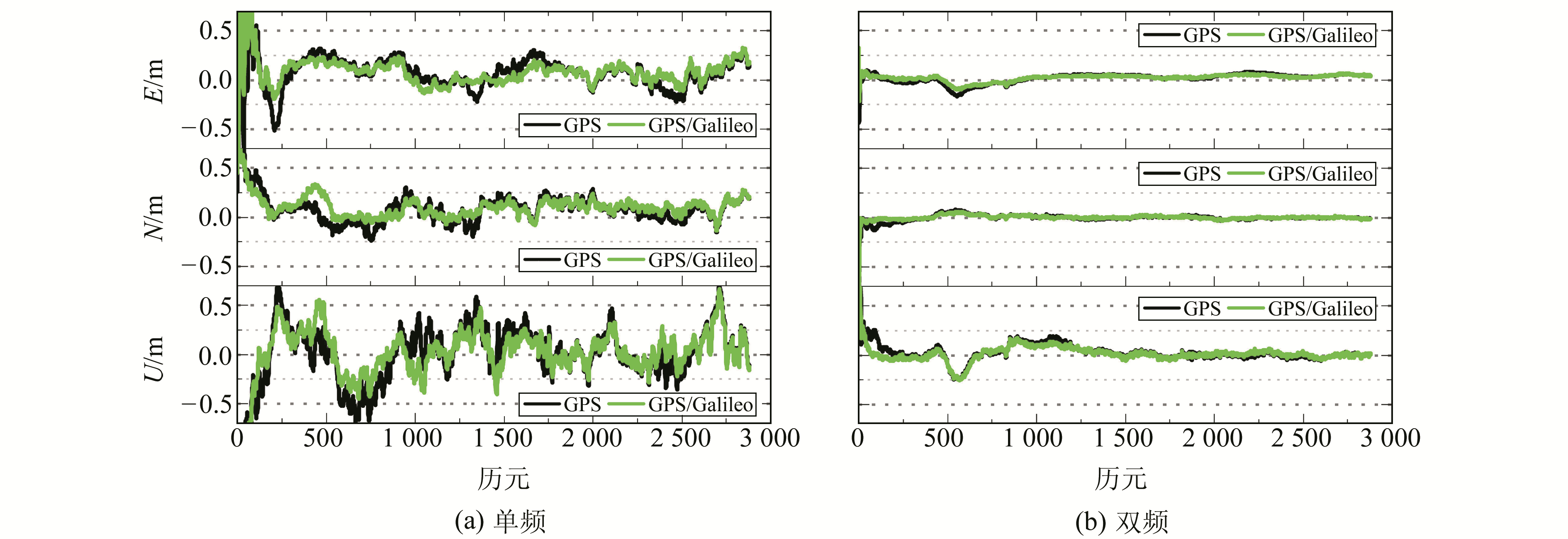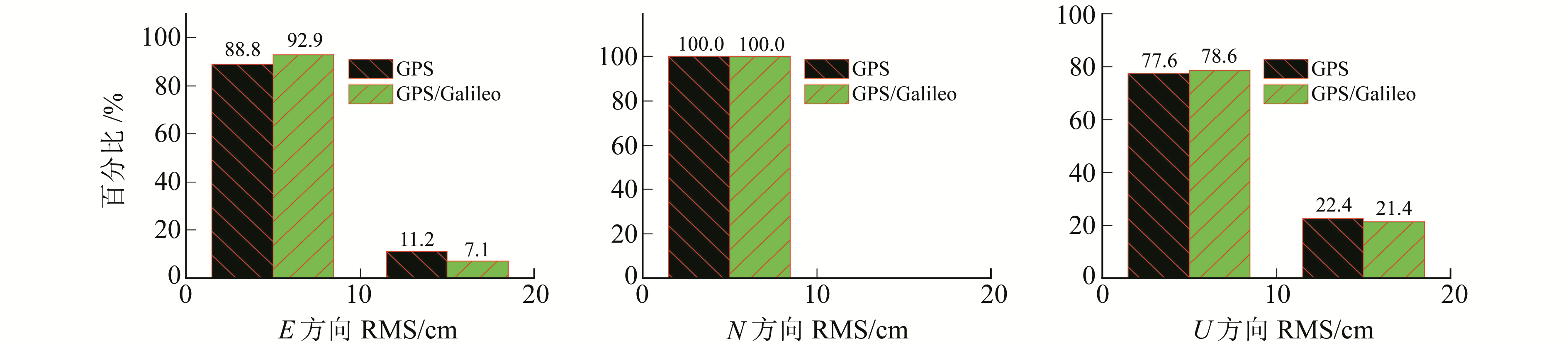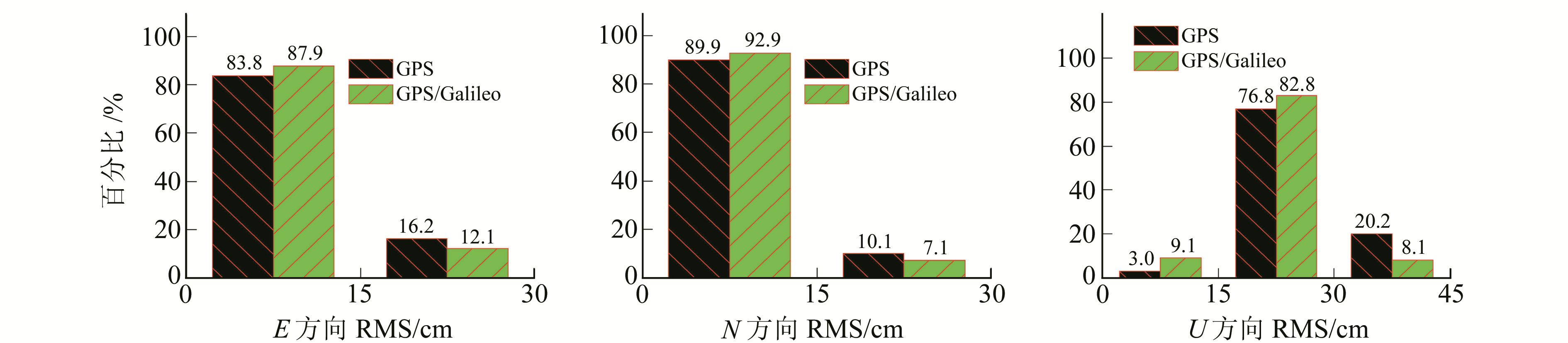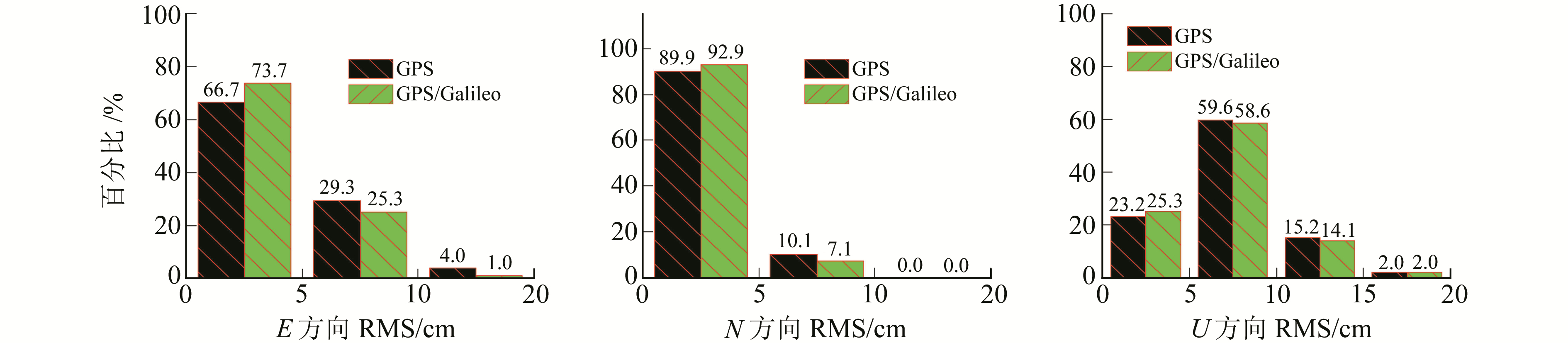﻿ GPS/Galileo实时精密单点定位精度分析文章快速检索 高级检索
 大地测量与地球动力学2019, Vol. 39Issue (8): 816-820  DOI: 10.14075/j.jgg.2019.08.010### 引用本文ZHAO Xingwang, GE Yulong. Accuracy Analysis of Real-Time GPS/Galileo Precise Point Positioning[J]. Journal of Geodesy and Geodynamics, 2019, 39(8): 816-820.### Foundation support

National Natural Science Foundation of China, No.41704008; Supporting Program for Excellent Young Talents in Colleges and Universities of Anhui Province, No.gxyq2017008; Youth Fund of Anhui University of Science and Technology, No.QN201512.

### 第一作者简介

ZHAO Xingwang, PhD, associate professor, majors in GNSS precision positioning method and its application, E-mail:xwzhao2008@126.com.

### 文章历史

GPS/Galileo实时精密单点定位精度分析
1. 安徽理工大学测绘学院，安徽省淮南市泰丰大街168号，232001;
2. 中国科学院国家授时中心，西安市书院东路3号，710600

1 精密单点定位模型

 $\begin{array}{c} P_{{\rm{r}}, j}^{\rm{s}} = \rho _{\rm{r}}^{\rm{s}} + c\cdot {\rm{d}}{t_{\rm{r}}} - c\cdot {\rm{d}}{t^{\rm{s}}} + {\rm{M}}{{\rm{F}}_{\rm{d}}}\left( e \right)\cdot {Z_{\rm{d}}} + \\ {\rm{M}}{{\rm{F}}_{\rm{w}}}\left( e \right)\cdot {Z_{\rm{w}}} + \gamma _j^{\rm{s}}\cdot I_{r, 1}^{\rm{s}} + ({\rm{d}}_{{\rm{r}}, j}^{\rm{s}} - {\rm{d}}_j^{\rm{s}}) + \varepsilon _{{\rm{r}}, j}^{\rm{s}}\left( P \right) \end{array}$ (1)
 $\begin{array}{l} \varPhi _{{\rm{r}}, j}^{\rm{s}} = \rho _r^{\rm{s}} + c\cdot {\rm{d}}{t_{\rm{r}}} - c\cdot {\rm{d}}{t^{\rm{s}}} + {\rm{M}}{{\rm{F}}_{\rm{d}}}\left( e \right)\cdot \\ {Z_{\rm{d}}} + {\rm{M}}{{\rm{F}}_{\rm{w}}}\left( e \right)\cdot {Z_{\rm{w}}} - \gamma _j^{\rm{s}}\cdot I_{r, 1}^{\rm{s}} + \lambda _j^{\rm{s}}\cdot \\ (N_{{\rm{r}}, j}^{\rm{s}} + b_{{\rm{r}}, j}^{\rm{s}} - b_j^{\rm{s}}) + \varepsilon _{{\rm{r}}, j}^{\rm{s}}\left( \varPhi \right) \end{array}$ (2)

 $\begin{array}{c} {P_{{\rm{IF}}}} = {\rm{ }}\frac{{f_1^2\cdot {P_1} - f_2^2\cdot {P_2}}}{{f_1^2 - f_2^2}} = \rho _{\rm{r}}^{\rm{s}} + c\cdot {\rm{d}}{t_{\rm{r}}} - \\ c\cdot {\rm{d}}{t^{\rm{s}}} + {\rm{M}}{{\rm{F}}_{\rm{d}}}\left( e \right)\cdot {Z_{\rm{d}}} + {\rm{M}}{{\rm{F}}_{\rm{w}}}\left( e \right)\cdot {Z_{\rm{w}}} + \\ (d_{{\rm{r, IF}}}^{\rm{s}} - d_{{\rm{IF}}}^{\rm{s}}) + \varepsilon _{\rm{r}}^{\rm{s}}({P_{{\rm{IF}}}}) \end{array}$ (3)
 $\begin{array}{l} {\varPhi _{{\rm{IF}}}} = {\rm{ }}\frac{{f_1^2\cdot {\varPhi _1} - f_2^2\cdot {\varPhi _2}}}{{f_1^2 - f_2^2}} = \rho _{\rm{r}}^{\rm{s}} + c\cdot {\rm{d}}{t_{\rm{r}}} - \\ c\cdot {\rm{d}}{t^{\rm{s}}} + {\rm{M}}{{\rm{F}}_{\rm{d}}}\left( e \right)\cdot {Z_{\rm{d}}} + {\rm{M}}{{\rm{F}}_{\rm{w}}}\left( e \right)\cdot {Z_{\rm{w}}} + \lambda _{{\rm{IF}}}^{\rm{s}}\cdot \\ (N_{{\rm{IF}}}^{\rm{s}} + b_{{\rm{r, IF}}}^{\rm{s}} - b_{{\rm{IF}}}^{\rm{s}}) + \varepsilon _{\rm{r}}^{\rm{s}}({\varPhi _{{\rm{IF}}}}) \end{array}$ (4)表 1 PPP数据处理策略 Tab. 1 PPP data processing strategy
2 实验与结果分析 2.1 实验说明

2.2 结果分析图 1 GPS和GPS/Galileo实时静态定位结果 Fig. 1 The positioning results of the real-time static GPS and GPS/Galileo PPP图 2 GPS和GPS/Galileo实时动态定位结果 Fig. 2 The positioning results of the real-time kinematic GPS and GPS/Galileo PPP图 3 单频实时静态PPP的RMS Fig. 3 RMS of the single-frequency real-time static PPP图 4 双频实时静态PPP的RMS Fig. 4 RMS of the dual -frequency real-time static PPP图 5 单频实时动态PPP的RMS Fig. 5 RMS of the single-frequency real-time kinematic PPP图 6 双频实时动态PPP的RMS Fig. 6 RMS of the dual-frequency real-time kinematic PPP表 2 实时静态PPP的平均RMS Tab. 2 The average RMS for the real-time static PPP表 3 实时动态PPP的平均RMS Tab. 3 The average RMS for the real-time kinematic PPP
3 结语

  Kouba J, Héroux P. Precise Point Positioning Using IGS Orbit and Clock Products[J]. GPS Solutions, 2001, 5(2): 12-28 DOI:10.1007/PL00012883 (0)  Li X X, Zhang X H, Ren X D, et al. Precise Positioning with Current Multi-Constellation Global Navigation Satellite Systems:GPS, GLONASS, Galileo and Beidou[J]. Scientific Reports, 2015(5): 8 328 (0)  Xia F Y, Ye S R, Xia P F, et al. Assessing the Latest Performance of Galileo-only PPP and the Contribution of Galileo to Multi-GNSS PPP[J]. Advances in Space Research, 2019, 63(9): 2 784-2 795 DOI:10.1016/j.asr.2018.06.008 (0)  Elsobeiey M, Al-Harbi S. Performance of Real-Time Precise Point Positioning Using IGS Real-Time Service[J]. GPS Solutions, 2015, 20(3): 565-571 (0)  Shi J B, Yuan X X, Cai Y, et al. GPS Real-Time Precise Point Positioning for Aerial Triangulation[J]. GPS Solutions, 2016, 21(2): 405-414 (0)  Kazmierski K, Hadas T, SoŠnica K. Weighting of Multi-GNSS Observations in Real-Time Precise Point Positioning[J]. Remote Sensing, 2018, 10(1): 84 (0)  佘忠伦, 高雅萍, 阿苦建英. 数据时效性对多系统实时PPP的影响分析[J]. 测绘科学, 2019, 44(2): 150-157 (She Zhonglun, Gao Yaping, Aku Jianying. The Influence Analysis of Multi-GNSS Real-Time PPP on Data Timeliness[J]. Science of Surveying and Mapping, 2019, 44(2): 150-157) (0)  张锡越, 赵春梅, 王权, 等. 多系统融合单频精密单点定位[J]. 测绘科学, 2018, 43(3): 29-34 (Zhang Xiyue, Zhao Chunmei, Wang Quan, et al. Precise Point Positioning with Multi-System Fusion for Single Frequency[J]. Science of Surveying and Mapping, 2018, 43(3): 29-34) (0)  Böhm J, Niell A, Tregoning P, et al. Global Mapping Function (GMF):A New Empirical Mapping Function Based on Numerical Weather Model Data[J]. Geophysical Research Letters, 2006, 33(7) (0)  Kouba J. A Guide to Using the IGS Products[Z]. Canada, 2015 https://kb.igs.org/hc/en-us/articles/201271873-A-Guide-to-Using-the-IGS-Products (0)  Zhao X W, Wang S L, Liu C, et al. Assessing the Performance of Multi-GNSS Precise Point Positioning in Asia-Pacific Region[J]. Survey Review, 2017, 49(354): 186-196 DOI:10.1080/00396265.2016.1151576 (0)  Cai C S, Gao Y, Pan L, et al. Precise Point Positioning with Quad-Constellations:GPS, Beidou, GLONASS and Galileo[J]. Advances in Space Research, 2015, 56(1): 133-143 DOI:10.1016/j.asr.2015.04.001 (0)  Guo F, Zhang X H, Wang J L. Timing Group Delay and Differential Code Bias Corrections for Beidou Positioning[J]. Journal of Geodesy, 2015, 89(5): 427-445 DOI:10.1007/s00190-015-0788-2 (0)  Ge Y L, Zhou F, Sun B Q, et al. The Impact of Satellite Time Group Delay and Inter-Frequency Differential Code Bias Corrections on Multi-GNSS Combined Positioning[J]. Sensors, 2017, 17(3): 602 DOI:10.3390/s17030602 (0)
Accuracy Analysis of Real-Time GPS/Galileo Precise Point Positioning
ZHAO Xingwang1GE Yulong2
1. School of Geomatics, Anhui University of Science and Technology, 168 Taifeng Street, Huainan 232001, China;
2. National Time Service Center, CAS, 3 East-Shuyuan Road, Xi'an 710600, China
Abstract: Based on analysis of combined precise point positioning (PPP) model, we investigate single- and dual-frequency real-time static and kinematic GPS and GPS/Galileo precise point positioning, using multi-GNSS real-time orbit and clock products released by Centre National d'Etudes Spatiales (CNES). Through the ten days observations from ten Multi-GNSS experiment (MGEX) stations, the solutions of different PPP models are then obtained. The results show that GPS/Galileo combined PPP outperforms GPS-only. However, while the accuracy of GPS/Galileo combined PPP is slightly better than GPS-only PPP for single- and dual-frequency real-time static modes, it presents a significant improvement for real-time kinematic mode. Compared with GPS-only PPP, the average accuracies of GPS/Galileo combined PPP are notably improved by 5.4%, 4.9% and 10.4%, which are 10.6 cm, 9.8 cm and 22.5 cm in the east, north and up directions, respectively, in the single-frequency kinematic positioning. Furthermore, the accuracies of dual-frequency real-time kinematic GPS/Galileo are about 4.3 cm, 2.9 cm and 7.0 cm in the E, N and U directions, respectively; these results present increases by 6.5%, 6.5% and 5.4% compared with GPS-only PPP. Moreover, the GPS/Galileo combination PPP has a certain improvement over the convergence time of a single GPS system.
Key words: real-time precise point positioning; single- and dual-frequency GPS/Galileo; real-time static and kinematic positioning; accuracy analysis